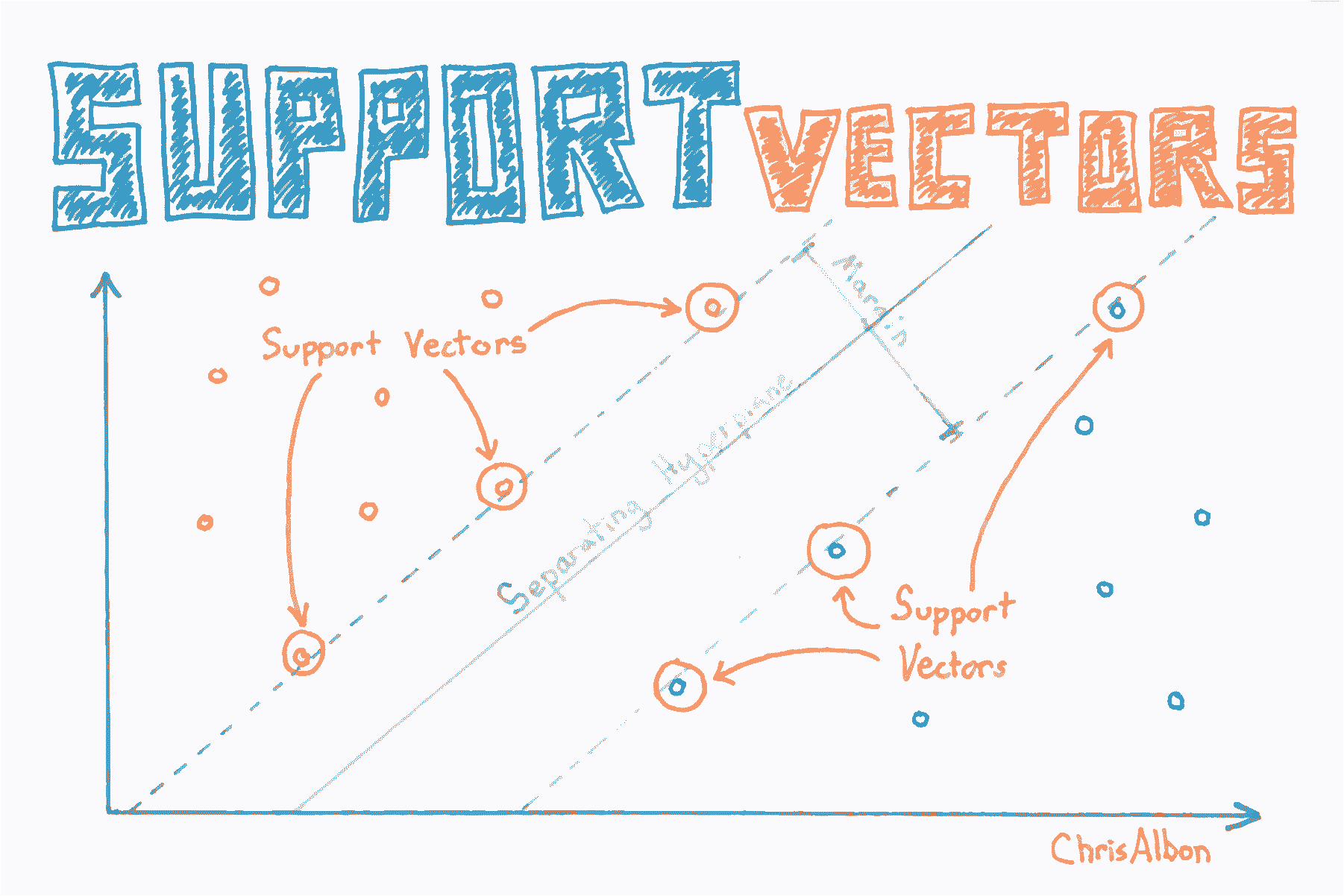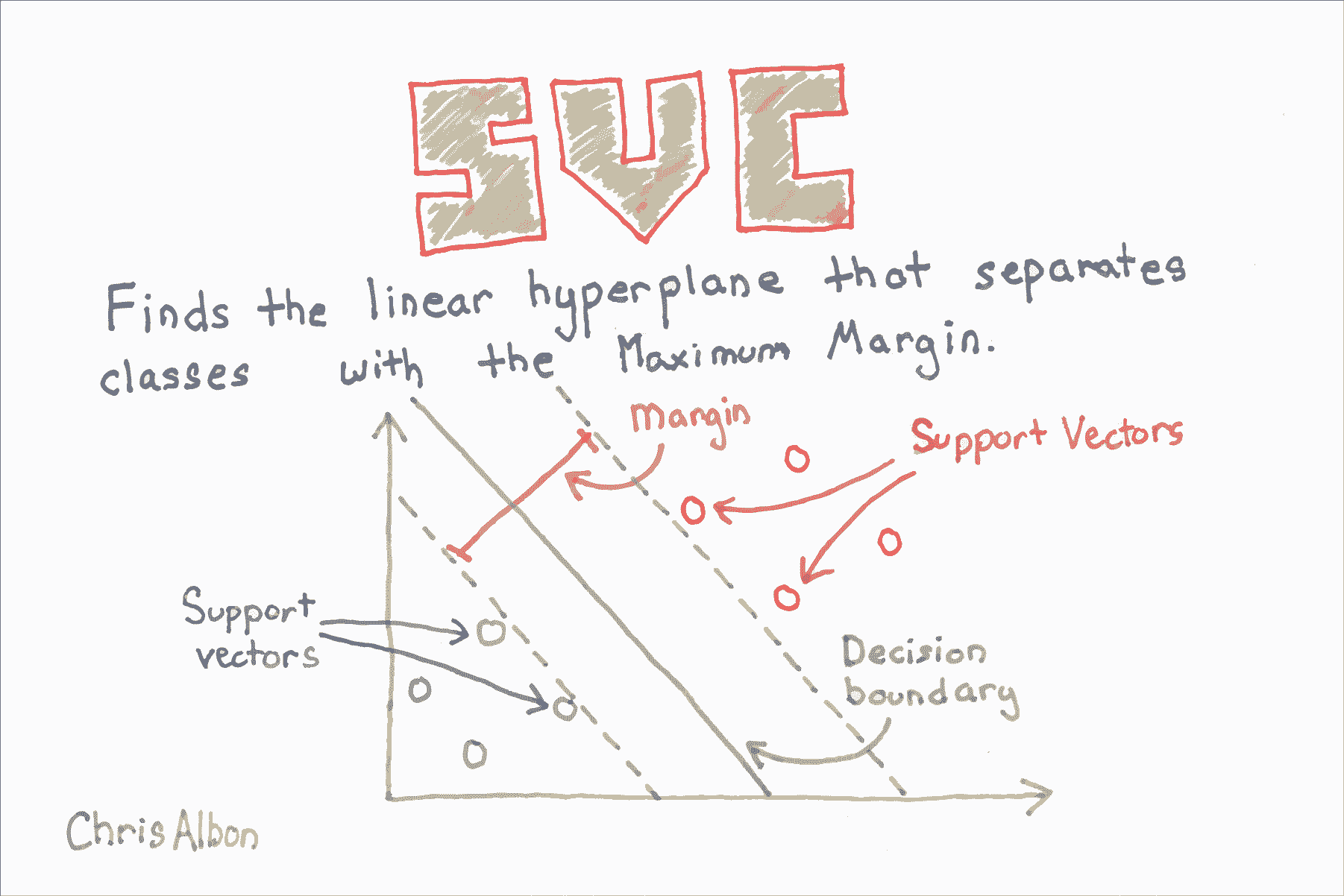# 十五、支持向量机

## 校准 SVC 中的预测概率

SVC 使用超平面来创建决策区域，不会自然输出观察是某一类成员的概率估计。 但是，我们实际上可以通过一些技巧输出校准的类概率。 在 SVC 中，可以使用 Platt 缩放，其中首先训练 SVC，然后训练单独的交叉验证逻辑回归来将 SVC 输出映射到概率：

$P(y=1 \mid x)={\frac {1}{1+e^{(A*f(x)+B)}}}$

# 加载库
from sklearn.svm import SVC
from sklearn import datasets
from sklearn.preprocessing import StandardScaler
import numpy as np

# 加载数据
X = iris.data
y = iris.target

# 标准化特征
scaler = StandardScaler()
X_std = scaler.fit_transform(X)

# 创建支持向量分类器对象
svc = SVC(kernel='linear', probability=True, random_state=0)

# 训练分类器
model = svc.fit(X_std, y)

# 创建新的观测
new_observation = [[.4, .4, .4, .4]]

# 查看预测的概率
model.predict_proba(new_observation)

# array([[ 0.00588822,  0.96874828,  0.0253635 ]])


## 寻找最近邻

# 加载库
from sklearn.neighbors import NearestNeighbors
from sklearn import datasets
from sklearn.preprocessing import StandardScaler
import numpy as np

# 加载数据
X = iris.data
y = iris.target


# 创建标准化器
standardizer = StandardScaler()

# 标准化特征
X_std = standardizer.fit_transform(X)

# 根据欧氏距离找到三个最近邻居（包括其自身）
nn_euclidean = NearestNeighbors(n_neighbors=3, metric='euclidean').fit(X)

# 列表的列表，表示每个观测的 3 个最近邻
nearest_neighbors_with_self = nn_euclidean.kneighbors_graph(X).toarray()

# 删除距离自身最近的一个观测
for i, x in enumerate(nearest_neighbors_with_self):
x[i] = 0

# 查看第一个观测的两个最近邻
nearest_neighbors_with_self

'''
array([ 0.,  0.,  0.,  0.,  1.,  0.,  0.,  0.,  0.,  0.,  0.,  0.,  0.,
0.,  0.,  0.,  0.,  1.,  0.,  0.,  0.,  0.,  0.,  0.,  0.,  0.,
0.,  0.,  0.,  0.,  0.,  0.,  0.,  0.,  0.,  0.,  0.,  0.,  0.,
0.,  0.,  0.,  0.,  0.,  0.,  0.,  0.,  0.,  0.,  0.,  0.,  0.,
0.,  0.,  0.,  0.,  0.,  0.,  0.,  0.,  0.,  0.,  0.,  0.,  0.,
0.,  0.,  0.,  0.,  0.,  0.,  0.,  0.,  0.,  0.,  0.,  0.,  0.,
0.,  0.,  0.,  0.,  0.,  0.,  0.,  0.,  0.,  0.,  0.,  0.,  0.,
0.,  0.,  0.,  0.,  0.,  0.,  0.,  0.,  0.,  0.,  0.,  0.,  0.,
0.,  0.,  0.,  0.,  0.,  0.,  0.,  0.,  0.,  0.,  0.,  0.,  0.,
0.,  0.,  0.,  0.,  0.,  0.,  0.,  0.,  0.,  0.,  0.,  0.,  0.,
0.,  0.,  0.,  0.,  0.,  0.,  0.,  0.,  0.,  0.,  0.,  0.,  0.,
0.,  0.,  0.,  0.,  0.,  0.,  0.])
'''


## 寻找支持向量# 加载库
from sklearn.svm import SVC
from sklearn import datasets
from sklearn.preprocessing import StandardScaler
import numpy as np

# 加载只有两个分类的数据
X = iris.data[:100,:]
y = iris.target[:100]

# 标准化特征
scaler = StandardScaler()
X_std = scaler.fit_transform(X)

# 创建支持向量对象
svc = SVC(kernel='linear', random_state=0)

# 训练分类器
model = svc.fit(X_std, y)

# 查看支持向量
model.support_vectors_

'''
array([[-0.5810659 ,  0.43490123, -0.80621461, -0.50581312],
[-1.52079513, -1.67626978, -1.08374115, -0.8607697 ],
[-0.89430898, -1.46515268,  0.30389157,  0.38157832],
[-0.5810659 , -1.25403558,  0.09574666,  0.55905661]])
'''

# 查看支持向量的下标
model.support_

# array([23, 41, 57, 98], dtype=int32)

# 查看每个分类的支持向量数
model.n_support_

# array([2, 2], dtype=int32)


## SVM 不平衡分类

$C_k = C * w_j$

$w_j = \frac{n}{kn_{j}}$

# 加载库
from sklearn.svm import SVC
from sklearn import datasets
from sklearn.preprocessing import StandardScaler
import numpy as np

# 加载只有两个类别的数据
X = iris.data[:100,:]
y = iris.target[:100]

# 通过删除前 40 个观察值使类高度不平衡
X = X[40:,:]
y = y[40:]

# 创建目标向量，表示类别是否为 0
y = np.where((y == 0), 0, 1)

# 标准化特征
scaler = StandardScaler()
X_std = scaler.fit_transform(X)

# 创建支持向量分类器
svc = SVC(kernel='linear', class_weight='balanced', C=1.0, random_state=0)

# 训练分类器
model = svc.fit(X_std, y)


## 绘制支持向量分类器超平面# 加载库
from sklearn.svm import LinearSVC
from sklearn import datasets
from sklearn.preprocessing import StandardScaler
import numpy as np
from matplotlib import pyplot as plt

# 加载只有两个分类和两个特征数据
X = iris.data[:100,:2]
y = iris.target[:100]

# 标准化特征
scaler = StandardScaler()
X_std = scaler.fit_transform(X)

# 创建支持向量分类器
svc = LinearSVC(C=1.0)

# 训练模型
model = svc.fit(X_std, y)


# 使用他们的类别绘制数据点和颜色
color = ['black' if c == 0 else 'lightgrey' for c in y]
plt.scatter(X_std[:,0], X_std[:,1], c=color)

# 创建超平面
w = svc.coef_
a = -w / w
xx = np.linspace(-2.5, 2.5)
yy = a * xx - (svc.intercept_) / w

# 绘制超平面
plt.plot(xx, yy)
plt.axis("off"), plt.show();## 使用 RBF 核时的 SVM 参数

# 导入可视化分类器的包
from matplotlib.colors import ListedColormap
import matplotlib.pyplot as plt
import warnings

# 导入执行分类的包
import numpy as np
from sklearn.svm import SVC


def versiontuple(v):
return tuple(map(int, (v.split("."))))

def plot_decision_regions(X, y, classifier, test_idx=None, resolution=0.02):

# 配置标记生成器和颜色表
markers = ('s', 'x', 'o', '^', 'v')
colors = ('red', 'blue', 'lightgreen', 'gray', 'cyan')
cmap = ListedColormap(colors[:len(np.unique(y))])

# 绘制决策平面
x1_min, x1_max = X[:, 0].min() - 1, X[:, 0].max() + 1
x2_min, x2_max = X[:, 1].min() - 1, X[:, 1].max() + 1
xx1, xx2 = np.meshgrid(np.arange(x1_min, x1_max, resolution),
np.arange(x2_min, x2_max, resolution))
Z = classifier.predict(np.array([xx1.ravel(), xx2.ravel()]).T)
Z = Z.reshape(xx1.shape)
plt.contourf(xx1, xx2, Z, alpha=0.4, cmap=cmap)
plt.xlim(xx1.min(), xx1.max())
plt.ylim(xx2.min(), xx2.max())

for idx, cl in enumerate(np.unique(y)):
plt.scatter(x=X[y == cl, 0], y=X[y == cl, 1],
alpha=0.8, c=cmap(idx),
marker=markers[idx], label=cl)

# 高亮测试样本
if test_idx:
# plot all samples
if not versiontuple(np.__version__) >= versiontuple('1.9.0'):
X_test, y_test = X[list(test_idx), :], y[list(test_idx)]
else:
X_test, y_test = X[test_idx, :], y[test_idx]

plt.scatter(X_test[:, 0],
X_test[:, 1],
c='',
alpha=1.0,
linewidths=1,
marker='o',
s=55, label='test set')


np.random.seed(0)
X_xor = np.random.randn(200, 2)
y_xor = np.logical_xor(X_xor[:, 0] > 0,
X_xor[:, 1] > 0)
y_xor = np.where(y_xor, 1, -1)

plt.scatter(X_xor[y_xor == 1, 0],
X_xor[y_xor == 1, 1],
c='b', marker='x',
label='1')
plt.scatter(X_xor[y_xor == -1, 0],
X_xor[y_xor == -1, 1],
c='r',
marker='s',
label='-1')

plt.xlim([-3, 3])
plt.ylim([-3, 3])
plt.legend(loc='best')
plt.tight_layout()
plt.show()# 使用线性核创建SVC分类器
svm = SVC(kernel='linear', C=1, random_state=0)
# 训练分类器
svm.fit(X_xor, y_xor)

# 可视化决策边界
plot_decision_regions(X_xor, y_xor, classifier=svm)
plt.legend(loc='upper left')
plt.tight_layout()
plt.show()$K(\mathbf {x} ,\mathbf {x’} )=\exp \left(-{\frac {||\mathbf {x} -\mathbf {x’} ||^{2}}{2\sigma ^{2}}}\right)$

### Gamma

gamma是 RBF 核的一个参数，可以被认为是核的“扩展”，因此也就是决策区域。 当gamma较低时，决策边界的“曲线”非常低，因此决策区域非常宽。 当gamma较高时，决策边界的“曲线”很高，这会在数据点周围创建决策边界的孤岛。 我们将在下面非常清楚地看到它。

### C

C是 SVC 学习器的参数，是对数据点的错误分类的惩罚。 当C很小时，分类器可以使用错误分类的数据点（高偏差，低方差）。 当C很大时，分类器因错误分类的数据而受到严重惩罚，因此与之相反来避免任何错误分类的数据点（低偏差，高方差）。

## Gamma

### Gamma = 0.01

# 使用 RBF 核创建 SVC 分类器
svm = SVC(kernel='rbf', random_state=0, gamma=.01, C=1)
# 训练分类器
svm.fit(X_xor, y_xor)

# 可视化决策边界
plot_decision_regions(X_xor, y_xor, classifier=svm)
plt.legend(loc='upper left')
plt.tight_layout()
plt.show()### Gamma = 1.0

# 使用 RBF 核创建 SVC 分类器
svm = SVC(kernel='rbf', random_state=0, gamma=1, C=1)
# 训练分类器
svm.fit(X_xor, y_xor)

# 可视化决策边界
plot_decision_regions(X_xor, y_xor, classifier=svm)
plt.legend(loc='upper left')
plt.tight_layout()
plt.show()### Gamma = 10.0

gamma = 10时，核的延展不太明显。 决策边界开始极大地受到各个数据点（即方差）的影响。

# 使用 RBF 核创建 SVC 分类器
svm = SVC(kernel='rbf', random_state=0, gamma=10, C=1)
# 训练分类器
svm.fit(X_xor, y_xor)

# 可视化决策边界
plot_decision_regions(X_xor, y_xor, classifier=svm)
plt.legend(loc='upper left')
plt.tight_layout()
plt.show()### Gamma = 100.0

# 使用 RBF 核创建 SVC 分类器
svm = SVC(kernel='rbf', random_state=0, gamma=100, C=1)
# 训练分类器
svm.fit(X_xor, y_xor)

# 可视化决策边界
plot_decision_regions(X_xor, y_xor, classifier=svm)
plt.legend(loc='upper left')
plt.tight_layout()
plt.show()## C - 惩罚参数

### C = 1

# 使用 RBF 核创建 SVC 分类器
svm = SVC(kernel='rbf', random_state=0, gamma=.01, C=1)
# 训练分类器
svm.fit(X_xor, y_xor)

# 可视化决策边界
plot_decision_regions(X_xor, y_xor, classifier=svm)
plt.legend(loc='upper left')
plt.tight_layout()
plt.show()### C = 10

C = 10时，分类器对错误分类的数据点的容忍度较低，因此决策边界更严格。

# 使用 RBF 核创建 SVC 分类器
svm = SVC(kernel='rbf', random_state=0, gamma=.01, C=10)
# 训练分类器
svm.fit(X_xor, y_xor)

# 可视化决策边界
plot_decision_regions(X_xor, y_xor, classifier=svm)
plt.legend(loc='upper left')
plt.tight_layout()
plt.show()### C = 1000

When C = 1000, the classifier starts to become very intolerant to misclassified data points and thus the decision boundary becomes less biased and has more variance (i.e. more dependent on the individual data points).

# 使用 RBF 核创建 SVC 分类器
svm = SVC(kernel='rbf', random_state=0, gamma=.01, C=1000)
# 训练分类器
svm.fit(X_xor, y_xor)

# 可视化决策边界
plot_decision_regions(X_xor, y_xor, classifier=svm)
plt.legend(loc='upper left')
plt.tight_layout()
plt.show()### C = 10000

C = 10000时，分类器“非常努力”，不会对数据点进行错误分类，我们会看到过拟合的迹象。

# 使用 RBF 核创建 SVC 分类器
svm = SVC(kernel='rbf', random_state=0, gamma=.01, C=10000)
# 训练分类器
svm.fit(X_xor, y_xor)

# 可视化决策边界
plot_decision_regions(X_xor, y_xor, classifier=svm)
plt.legend(loc='upper left')
plt.tight_layout()
plt.show()### C = 100000

C = 100000时，对于任何错误分类的数据点，分类器都会受到严重惩罚，因此边距很小。

# 使用 RBF 核创建 SVC 分类器
svm = SVC(kernel='rbf', random_state=0, gamma=.01, C=100000)
# 训练分类器
svm.fit(X_xor, y_xor)

# 可视化决策边界
plot_decision_regions(X_xor, y_xor, classifier=svm)
plt.legend(loc='upper left')
plt.tight_layout()
plt.show()## 支持向量分类器SVC 在最大化超平面边距和最小化错误分类之间取得平衡。 在 SVC 中，后者由超参数 $C$ 控制，对错误施加惩罚。C是 SVC 学习器的参数，是对数据点进行错误分类的惩罚。 当C很小时，分类器可以使用错误分类的数据点（高偏差但低方差）。 当C很大时，分类器因错误分类的数据而受到严重惩罚，因此向后弯曲避免任何错误分类的数据点（低偏差但高方差）。

# 加载库
from sklearn.svm import LinearSVC
from sklearn import datasets
from sklearn.preprocessing import StandardScaler
import numpy as np

# 加载特征和目标数据
X = iris.data
y = iris.target

# 标准化特征
scaler = StandardScaler()
X_std = scaler.fit_transform(X)

# 创建支持向量分类器
svc = LinearSVC(C=1.0)

# 训练模型
model = svc.fit(X_std, y)

# 创建新的观测
new_observation = [[-0.7, 1.1, -1.1 , -1.7]]

# 预测新观测的类别
svc.predict(new_observation)

# array()


08-131214

#### 支持向量机

09-286万+

#### 简单粗暴理解支持向量机（SVM）及其MATLAB实例07-029573

#### Python 数据科学手册 5.7 支持向量机

11-083455

#### 支持向量机 SVM 算法推导优缺点 代码实现 in Python

04-272028

#### 【机器学习实战】支持向量机----分类库和简单训练mnist

08-181589

#### 《机器学习实战》学习笔记（六）：支持向量机

12-072328

#### 稀疏化鲁棒最小二乘支持向量机与多目标遗传优化

12-189740

#### 结构风险最小化(SRM)

06-27394

#### 一个简单的案例带你了解支持向量机算法（Python代码）

02-14680

#### 从示例中了解svm(支持向量机)算法（以及代码）

04-011131

#### 【机器学习算法笔记系列】支持向量机(SVM)算法详解和实战

11-011298

#### 半监督学习（五）——半监督支持向量机

05-282万+

#### 开发者自述：我是怎样理解支持向量机（SVM）与神经网络的

11-081314

#### 基于卷积神经网络（cnn)与支持向量机(svm)结合的猫狗识别

04-191440

#### 考察数据科学家支持向量机（SVM）知识的25道题，快来测测吧

06-213678

#### 笔记︱支持向量机SVM在金融风险欺诈中应用简述

05-21579

#### 机器学习算法---支持向量机

10-27335

#### 支持向量机实例讲解

02-1928万+

#### 软件测试入门、SQL、性能测试、测试管理工具

03-02©️2020 CSDN 皮肤主题: 黑客帝国 设计师: 上身试试点击重新获取扫码支付1.余额是钱包充值的虚拟货币，按照1:1的比例进行支付金额的抵扣。
2.余额无法直接购买下载，可以购买VIP、C币套餐、付费专栏及课程。余额充值